GeeksforGeeks App
Open AppBrowser
Continue

# Reshaping Pandas Dataframes using Melt And Unmelt

Pandas is an open-source, BSD-licensed library written in Python Language. Pandas provide high performance, fast, easy to use data structures and data analysis tools for manipulating numeric data and time series. Pandas is built on the Numpy library and written in languages like Python, Cython, and C. In 2008, Wes McKinney developed the Pandas library. In pandas, we can import data from various file formats like JSON, SQL, Microsoft Excel, etc. The dataframes feature is used to load and do manipulations on the data.

Sometimes we need to reshape the Pandas data frame to perform analysis in a better way. Reshaping plays a crucial role in data analysis. Pandas provide function like melt and unmelt for reshaping.

## Pandas.melt()

melt() is used to convert a wide dataframe into a longer form. This function can be used when there are requirements to consider a specific column as an identifier.

Syntax: pandas.melt(frame, id_vars=None, value_vars=None, var_name=None, value_name=’value’, col_level=None)

Example 1:

Initialize the dataframe with data regarding ‘Days‘, ‘Patients‘ and ‘Recovery‘.

## Python3

 `# importing pandas library``import` `pandas as pd` `# creating and initializing a list``values ``=` `[[``'Monday'``, ``65000``, ``50000``],``          ``[``'Tuesday'``, ``68000``, ``45000``],``          ``[``'Wednesday'``, ``70000``, ``55000``],``          ``[``'Thursday'``, ``60000``, ``47000``],``          ``[``'Friday'``, ``49000``, ``25000``],``          ``[``'Saturday'``, ``54000``, ``35000``],``          ``[``'Sunday'``, ``100000``, ``70000``]]` `# creating a pandas dataframe``df ``=` `pd.DataFrame(values, columns``=``[``'DAYS'``, ``'PATIENTS'``, ``'RECOVERY'``])` `# displaying the data frame``df`

Output: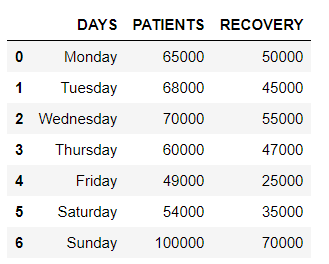Now, we reshape the data frame using pandas.melt() around column ‘DAYS‘.

## Python3

 `# melting with DAYS as column identifier``reshaped_df ``=` `df.melt(id_vars``=``[``'DAYS'``])` `# displaying the reshaped data frame``reshaped_df`

Output: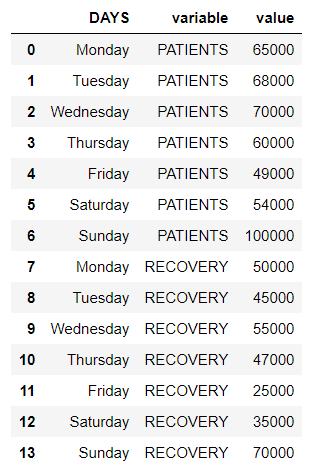Example 2:

Now, to the dataframe used above a new column named ‘Deaths‘ is introduced.

## Python3

 `# importing pandas library``import` `pandas as pd` `# creating and initializing a dataframe``values ``=` `[[``'Monday'``, ``65000``, ``50000``, ``1500``],``          ``[``'Tuesday'``, ``68000``, ``45000``, ``7250``],``          ``[``'Wednesday'``, ``70000``, ``55000``, ``1400``],``          ``[``'Thursday'``, ``60000``, ``47000``, ``4200``],``          ``[``'Friday'``, ``49000``, ``25000``, ``3000``],``          ``[``'Saturday'``, ``54000``, ``35000``, ``2000``],``          ``[``'Sunday'``, ``100000``, ``70000``, ``4550``]]` `# creating a pandas dataframe``df ``=` `pd.DataFrame(values,``                  ``columns``=``[``'DAYS'``, ``'PATIENTS'``, ``'RECOVERY'``, ``'DEATHS'``])` `# displaying the data frame``df`

Output: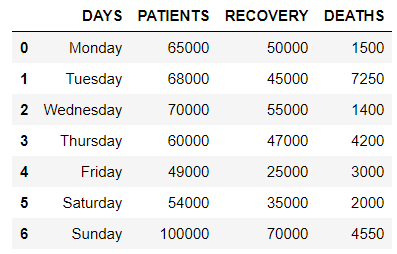we reshaped the data frame using pandas.melt() around column ‘PATIENTS‘.

## Python3

 `# reshaping data frame``# using pandas.melt()``reshaped_df ``=` `df.melt(id_vars``=``[``'PATIENTS'``])` `# displaying the reshaped data frame``reshaped_df`

Output: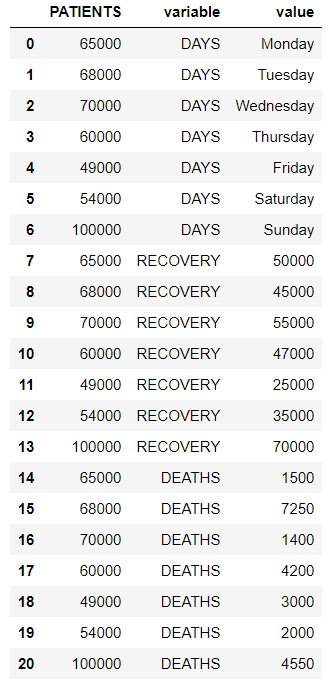## Pandas.pivot()/ unmelt function

Pivoting, Unmelting or Reverse Melting is used to convert a column with multiple values into several columns of their own.

Syntax : DataFrame.pivot(index=None, columns=None, values=None)

Example 1:

Create a dataframe that contains the data on ID, Name, Marks and Sports of 6 students.

## Python3

 `# importing pandas library``import` `pandas as pd` `# creating and initializing a list``values ``=` `[[``101``, ``'Rohan'``, ``455``, ``'Football'``],``          ``[``111``, ``'Elvish'``, ``250``, ``'Chess'``],``          ``[``192``, ``'Deepak'``, ``495``, ``'Cricket'``],``          ``[``201``, ``'Sai'``, ``400``, ``'Ludo'``],``          ``[``105``, ``'Radha'``, ``350``, ``'Badminton'``],``          ``[``118``, ``'Vansh'``, ``450``, ``'Badminton'``]]` `# creating a pandas dataframe``df ``=` `pd.DataFrame(values,``                  ``columns``=``[``'ID'``, ``'Name'``, ``'Marks'``, ``'Sports'``])` `# displaying the data frame``df`

Output: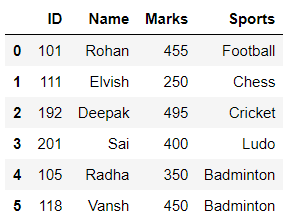Unmelting around the column Sports:

## Python3

 `# unmelting``reshaped_df ``=` `df.pivot(index``=``'Name'``, columns``=``'Sports'``)` `# displaying the reshaped data frame``reshaped_df`

Output: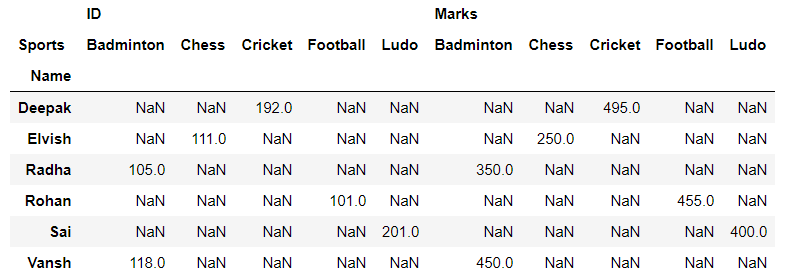Example 2:

Consider the same dataframe used in the example above. Unmelting can be done based on more than one column also.

## Python3

 `reshaped_df ``=` `df.pivot(``'ID'``, ``'Marks'``, ``'Sports'``)` `# displaying the reshaped data frame``reshaped_df`

Output: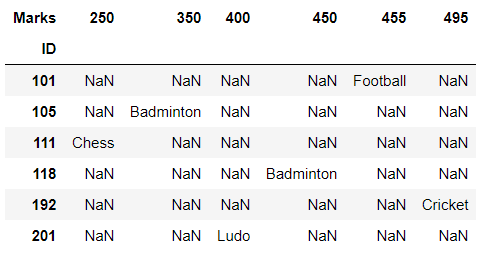But the reshaped dataframe appears little different from the original one in terms of index. To get the index also set as original dataframe use reset_index() function on the reshaped dataframe.

## Python3

 `reshaped_df ``=` `df.pivot(``'ID'``, ``'Marks'``, ``'Sports'``)` `# resetting index``df_new ``=` `reshaped_df.reset_index()` `# displaying the reshaped data frame``df_new`

Output: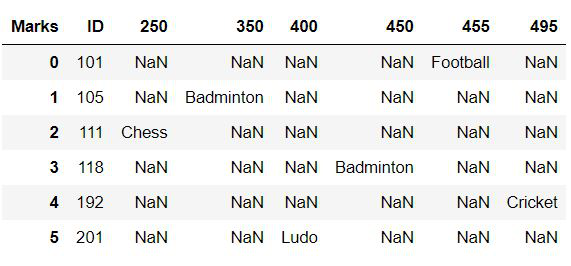My Personal Notes arrow_drop_up